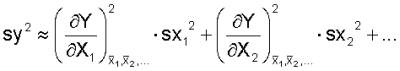## Error Progagation

If an unknown quantity is to be calculated from a function of normally distributed independent random variables its uncertainty (variance) can be calculated by the law of error propagation:For basic arithmetical operation it looks like this:The method of error propagation is part of the algorithm for data reconciliation that is performed automatically when calculating with STAN.